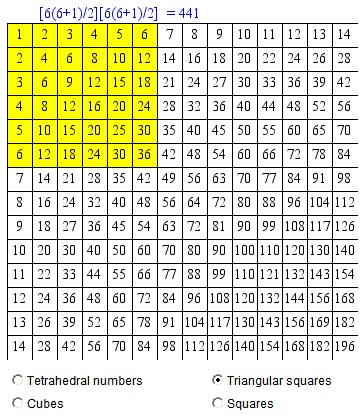# Examples with series of figurate numbers

There are many patterns in the common multiplication table. Some are suggested by the applet below.

### If you are reading this, your browser is not set to run Java applets. Try IE11 or Safari and declare the site https://www.cut-the-knot.org as trusted in the Java setup.What if applet does not run?

Just move the mouse over.

Discussion### Identities in the Multiplication Table

The applet points to four identities:

 (1) (1 + 2 + 3 + ... + n)2 = [n(n+1)/2]2 (2) 2n(1 + 2 + 3 + ... + n) - n2 = n3 (3) 12 + 22 + 32 + ... + n2 = n(n + 1)(2n + 1)/6 (4) n·1 + (n - 1)·2 + (n - 2)·3 + ... + 2·(n - 1) + 1·n = n(n + 1)(n + 2)/6The sum of all entries in an n×n multiplication table equals [n(n+1)/2]2. Indeed, the sum of the entries in the first row is 1 + 2 + ... + n = n(n + 1)/2. Then grouping the entries by rows be get

Row Sum 1 1 + 2 + ... + n = 1·n(n + 1)/2 2 2 + 4 + ... + 2n = 2·n(n + 1)/2 3 3 + 6 + ... + 3n = 3·n(n + 1)/2 ... n n + 2n + ... + n·n = n·n(n + 1)/2 Total (1 + 2 + ... + n)·n(n + 1)/2 = [n(n+1)/2]2

This settles (1).(2) arises as the sum of entries in the nth column and in the nth row, the diagonal term n2 being counted only once. The entries in both the nth row and the nth column add up to

n·(1 + 2 + ... + n) = n2(n + 1)/2.

Together, but with the exclusion of the diagonal entry, we get

2·n2(n + 1)/2 - n2 = (n3 + n2) - n2 = n3.

As a bonus, a combination of (1) and (2) leads to the following curious identity, known as Nicomachus' theorem

(1 + 2 + ... + n)2 = 13 + 23 + ... + n3.(3) is the sum of the diagonal entries, i.e., the sum of the first n squares. The formula is well known and is easily proven by induction. Mathematical induction, however, though a powerful tool, is limited to the cases when the formula to be proven is known (or has been guessed) up front. But how does one get those formulas in the first place? Here is one possible approach based on the theory of generating functions.

Denote qn = 12 + 22 + 32 + ... + n2. Then obviously the sequence {qn} satisfies the recurrence relation

qn = qn-1 + n2, q0 = 0.

Multiply the relation by xn-1 and add up the first n:

Q(x)/x = Q(x) + ∑nn2xn-1,

where Q(x) is the generating function of the sequence {qn}. This would be determined from

 (5) Q(x)(1 - x)/x = ∑nn2xn-1

if we could express the sum on the right in terms of x. Let's try,

nn2xn = ∑nn(n + 1)xn-1 - ∑nnxn-1

The sum ∑nnxn-1 has been found to equal 1/(1 - x)2 as the formal derivative of the sum of the geometric series 1/(1 - x) = 1 + x + x2 + ... We shall apply the same idea to the series ∑nn(n + 1)xn-1.

 ∑nn(n + 1)xn-1 = [1/(1 - x)]'' = [1/(1 - x)2]' = 2/(1 - x)3.

From (5) then

 Q(x) = x/(1 - x)·(2/(1 - x)3 - 1/(1 - x)2) = x/(1 - x)·(1 + x)/(1 - x)3 = x(1 + x)/(1 - x)4.

We know thatnC(n,k)xn = xk/(1-x)k+1, which implies

 [xn]Q(x) = [xn]x/(1 - x)4 + [xn]x2/(1 - x)4 = C(n+2,3) + C(n+1,3) = (n + 2)(n + 1)n/6 + (n + 1)n(n - 1)/6 = n(n + 1)(2n + 1)/6.In (4), the coefficients are obtained by convolution of the terms of the plain sequence {n}. Convolutions arise when power series are multiplied term by term according to the definition. Let t0 = 0 and t1 = 0 and, for n = 2, 3, ...,, define tn = (n - 1)·1 + (n - 2)·2 + (n - 3)·3 + ... + 2·(n - 2) + 1·(n - 1). Introduce the generating function T(x) = ∑ntnxn.

 T(x) = (x + 2x2 + 3x3 + ...)·(x + 2x2 + 3x3 + ...) = x/(1 - x)2·x/(1 - x)2 = x2/(1 - x)4.

Using the same identity as above,

[xn]T(x) = [xn]x2/(1 - x)4 = C(n+1,3) = (n + 1)n(n - 1)/6.

Thus tn = (n + 1)n(n - 1)/6, which corresponds to the sum of terms in the (n - 1)st diagonal.### Generating Functions